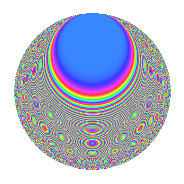# Properties

 Label 320.6.oLevel 320 Weight 6 Character orbit o Rep. character $$\chi_{320}(223,\cdot)$$ Character field $$\Q(\zeta_{4})$$ Dimension 120 Sturm bound 288

# Related objects

## Defining parameters

 Level: $$N$$ $$=$$ $$320 = 2^{6} \cdot 5$$ Weight: $$k$$ $$=$$ $$6$$ Character orbit: $$[\chi]$$ $$=$$ 320.o (of order $$4$$ and degree $$2$$) Character conductor: $$\operatorname{cond}(\chi)$$ $$=$$ $$40$$ Character field: $$\Q(i)$$ Sturm bound: $$288$$

## Dimensions

The following table gives the dimensions of various subspaces of $$M_{6}(320, [\chi])$$.

Total New Old
Modular forms 504 120 384
Cusp forms 456 120 336
Eisenstein series 48 0 48

## Trace form

 $$120q + O(q^{10})$$ $$120q + 2424q^{17} + 18696q^{25} - 106104q^{65} + 30216q^{73} - 1325400q^{81} - 1275480q^{97} + O(q^{100})$$

## Decomposition of $$S_{6}^{\mathrm{new}}(320, [\chi])$$ into newform subspaces

The newforms in this space have not yet been added to the LMFDB.

## Decomposition of $$S_{6}^{\mathrm{old}}(320, [\chi])$$ into lower level spaces

$$S_{6}^{\mathrm{old}}(320, [\chi]) \cong$$ $$S_{6}^{\mathrm{new}}(40, [\chi])$$$$^{\oplus 4}$$$$\oplus$$$$S_{6}^{\mathrm{new}}(160, [\chi])$$$$^{\oplus 2}$$

## Hecke characteristic polynomials

There are no characteristic polynomials of Hecke operators in the database